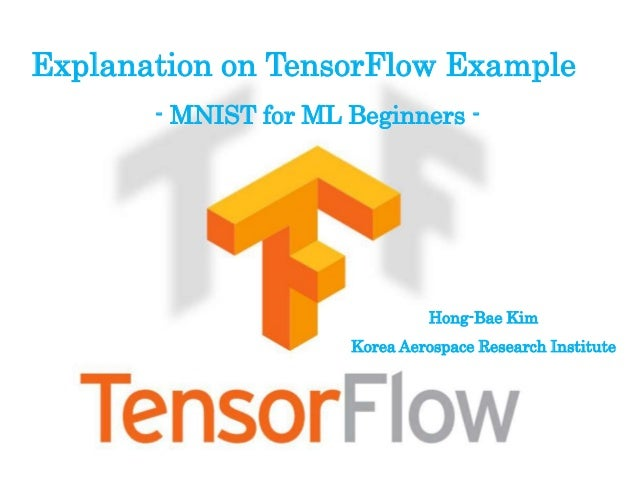Successfully reported this slideshow.Upcoming SlideShare
×

# MNIST for ML beginners

4,093 views

Published on

Tensorflow Tutorial 예제 중 ML 입문자들을 위한 예제. 간단한 Classifier Nets을 구성하여 Nets의 훈련 및 검증과정을 배울 수 있는 예제

Published in: Engineering
• Full Name
Comment goes here.

Are you sure you want to Yes No### MNIST for ML beginners

1. 1. Explanation on TensorFlow Example - MNIST for ML Beginners - Hong-Bae Kim Korea Aerospace Research Institute
2. 2. x_image (28x28) Reshape 28x28  784x1 vector . . . 10 digits Networks Architecture . . W, bx y=softmax(Wx+b) Classifier Nets Very simple Classier Nets
3. 3. MNIST For ML Beginners • Machine Learning 입문자를 위한 손글씨 숫자 분류기 만들기 • MNIST는 간단한 이미지의 집합으로 아래와 같은 손으로 적은 숫자로 구성 • 간단한 Classifier Nets를 구성하고 작동원리를 이해 • Softmax Regression으로 숫자를 추정
4. 4.  Define phase : Computation result is not determined  Define data and model  Construct learning model  Define cost function and optimizer  Run phase : can get a computation result in the case of putting model into session  Execute computation  Learning process using optimizer To execute the graph, Needs to connect with Core module Real computation is performed in Core module Computation process consists of two phases
5. 5. • Tensorflow의 라이브러리를 불러옴. >>> import tensorflow as tf • MNIST 데이터 다운로드 >>> import input_data >>> mnist = input_data.read_data_sets('MNIST_data', one_hot=True) mnist.tran：training 데이터 55,000 mnist.test：test 데이터 10,000 mnist.validation：validation 데이터 5,000 >>> sess = tf.InteractiveSession() 데이터 불러오기 Define Phase
6. 6. mnist.trans.xs, mnist.trans.ys 를 아래와 같이 정의 각이미지의 출력정답데이터 28pixel×28pixel의 이미지를 28×28=784의 벡터로 변환 벡터의 요소는 0(백)~1(흑)의 실수 각이미지의 입력벡터 데이터 1 0 0 0 0 0 0 0 0 0 “0”일 경우 0 0 0 0 0 1 0 0 0 0 “5”일 경우 Define Phase
7. 7. 변수의 정의 • 입력 이미지데이터(28x28=784)의 텐서 사이즈를 정의 >>> x = tf.placeholder(tf.float32, [None, 784]) • Weight 사이즈를 정의하고 초기화 >>> W = tf.Variable(tf.zeros([784, 10])) • Bias의 사이즈를 정의하고 초기화 >>> b = tf.Variable(tf.zeros()) • 출력의 정의 >>> y = tf.nn.softmax(tf.matmul(x, W) + b) • 출력 정답데이터(10x1)의 텐서 사이즈를 정의 >>> y_ = tf.placeholder(tf.float32, [None, 10]) Define Phase
8. 8. Softmax Regressions 입력데이터(x)가 Nets의 연산과정(Wx+b)을 거친 후 10개의 숫자 중 어느 것에 해당하는지에 대한 확률 계산 𝑝 𝑦(𝑖) = 0 𝑥(𝑖); 𝑤 ⋮ 𝑝 𝑦(𝑖) = 9 𝑥(𝑖); 𝑤 = 1 𝑗=1 10 e 𝑤j T 𝑥(𝑖) ew1 T 𝑥(𝑖) ⋮ ew10 T 𝑥(𝑖) y=softmax(Wx+b) 0.85 0.05 0.05 0.01 0.01 0.01 0.01 0.02 0.01 0.03 “0”일 경우 0.01 0.05 0.05 0.01 0.01 0.90 0.01 0.02 0.01 0.03 “5”일 경우 ∑=1 ∑=1 Define Phase
9. 9. 손실함수(Loss Function)의 정의 >>> cross_entropy = -tf.reduce_sum(y_*tf.log(y)) For 𝑦_𝑖 = 1 𝑐𝑎𝑠𝑒 J(w)=log𝑦𝑖 𝑦𝑖 1 J(w) As 𝑦𝑖 approaches to 1, J(w) becomes 0 J(w)=-∑𝑦_𝑖•log𝑦𝑖 y : 분류기에서 추정한 확률값 y_ : 정답 Define Phase
10. 10. >>> train_step = tf.train.GradientDescentOptimizer(0.01).minimize(cross_entropy) Backpropagation Algorithm의 정의 cross_entropy를 최소화하도록 GradientDescentOptimizer를 사용하여 훈련한다. Define Phase Learning rate
11. 11. Run phase # 변수 초기화 >>> init = tf.initialize_all_variables() >>> sess = tf.Session() >>> sess.run(init) 훈련실시 >>> for i in range(1000): ... batch_xs, batch_ys = mnist.train.next_batch(100) ... sess.run(train_step, feed_dict={x: batch_xs, y_: batch_ys}) i<1000 ? 랜덤하게 100개의 이미지 데이터 를 선택하여 입력을 batch_xs, 출력정답을 batch_ys로 지정 훈련 실시 i=i+1 yes No end
12. 12. Run phase 훈련된 분류기의 검증 • 정답의 정의 >>> correct_prediction = tf.equal(tf.argmax(y, 1), tf.argmax(y_, 1)) Returns the index with the largest value across dimensions of a tensor Return “1” if argmax(y, 1) = argmax(y_, 1), otherwise return “0” • 정확도의 정의 >>> accuracy = tf.reduce_mean(tf.cast(correct_prediction, 'float')) Casts a tensor to “float” calculate mean value • 정답율을 계산하여 표시 >>> print(accuracy.eval({x: mnist.test.images, y_: mnist.test.labels}))